# How to Get the Maximum or Minimum Absolute Value in Excel

It is easy to find the maximum or minimum value in a batch of data in excel, but if this batch of numbers contains both positive and negative numbers, the maximum or minimum absolute value cannot be found out by basic MAX or MIN function in excel. This article will help you to solve this issue by using ABS function.

Prepare a table of numbers contains both positive and negative numbers: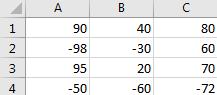As we all know, compare the absolute values for positive number and negative number, actually we compare the values without negative sign, so`|-11|` is larger than `|10|`. How can we find out the maximum or minimum value among the positive and negative numbers? Please follow below steps.

## Get the Maximum or Minimum Absolute Value in Excel

Step 1: Select a blank cell, enter the formula =MAX(ABS(A1:C4)).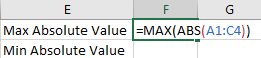Step 2: Press Ctrl + Shift + Enter together, then the MAX absolute value is displayed.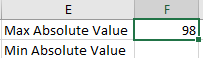Step 3: Use the similar formula to get the MIN absolute value. Enter the formula =MIN(ABS(A1:C4)).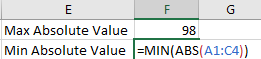Step 4: Press Ctrl + Shift + Enter together, then the MIN absolute value is displayed.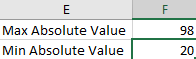## Get the Maximum or Minimum Absolute Value by User Defined Function

We can also define a function like MINABS or MINABS then we can directly use it to find out the MAX or MIN absolute value. See steps below.

Step 1: Click Developer tab->Visual Basic or Alt+F11 to load Microsoft Visual Basic for Applications window.

Step 2: Click Insert->Module to insert a module.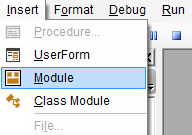Step 3: Enter below code in Module window.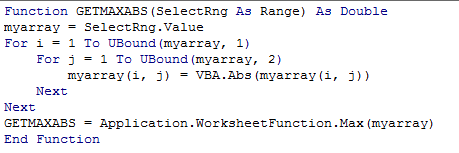```Function GETMAXABS(SelectRng As Range) As Double
myarray = SelectRng.Value
For i = 1 To UBound(myarray, 1)
For j = 1 To UBound(myarray, 2)
myarray(i, j) = VBA.Abs(myarray(i, j))
Next
Next
GETMAXABS = Application.WorksheetFunction.Max(myarray)
End Function```

Notes:

1. We define the function GETMAXABS to get the maximum absolute value in excel.
2. We can use the similar code to define another function GETMINABS to get the minimum absolute value as well. Just replace ‘max’ to ‘min’ in above code.

Step 4: Now we can try the user defined function GETMAXABS. After entering =get, we can find that defined function GETMAXABS is loaded properly.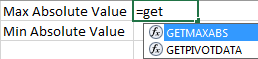Step 5: Enter =GETMAXABS(A1:C4).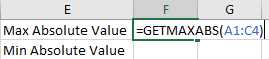Step 6: This time click Enter. Verify that MAX absolute value is displayed.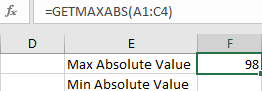### Related Functions

• Excel ABS Function
The Excel ABS function returns the absolute value of a number.The ABS function is a build-in function in Microsoft Excel and it is categorized as a Math and Trigonometry Function.The syntax of the ABS function is as below:=ABS (number)…
• Excel MIN function
The Excel MIN function returns the smallest numeric value from the numbers that you provided. Or returns the smallest value in the array.The MIN function is a build-in function in Microsoft Excel and it is categorized as a Statistical Function.The syntax of the MIN function is as below:= MIN(num1,[num2,…numn])….
• Excel MAX function
The Excel MAX function returns the largest numeric value from the numbers that you provided. Or returns the largest value in the array.= MAX(num1,[num2,…numn])…

Sidebar